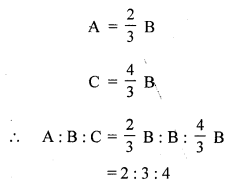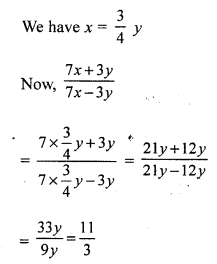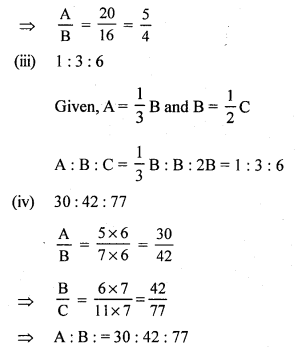# RS Aggarwal Class 7 Solutions Chapter 8 Ratio and Proportion CCE Test Paper

In this chapter, we provide RS Aggarwal Solutions for Class 7 Chapter 8 Ratio and Proportion CCE Test Paper for English medium students, Which will very helpful for every student in their exams. Students can download the latest RS Aggarwal Solutions for Class 7 Chapter 8 Ratio and Proportion CCE Test Paper Maths pdf, free RS Aggarwal Solutions Class 7 Chapter 8 Ratio and Proportion CCE Test Paper Maths book pdf download. Now you will get step by step solution to each question.

### RS Aggarwal Solutions for Class 7 Chapter 8 Ratio and Proportion CCE Test Paper Download PDF

Question 1.
Solution:Question 2.
Solution:
The sum of ratio terms is = 2 + 3 + 5 = 10
Then, we have :
A’s share = ₹ 210 x 1100 = ₹ 220
B’s share = ₹ 310 x 1100 = ₹ 330
C’s share = ₹ 510 x 1100 = ₹ 550

Question 3.
Solution:
Product of the extremes = 25 x 6 = 150
Product of the means = 36 x 5 = 180
The product of the extremes is not equal to that of the means.
Hence, 25, 36, 5 and 6 are not in proportion.

Question 4.
Solution:
x : 18 :: 18 : 108
⇒ x x 108 = 18 x 18
(Product of extremes = Product of means)
⇒ 108x = 324
⇒ x = 3
Hence, the value of x is 3

Question 5.
Solution:
Suppose that the numbers are 5x and 7x
Then, 5x + 7x = 84
12x = 84
x = 7
Hence, the numbers are (5 x 7) = 35 and (7 x 7) = 49.

Question 6.
Solution:
Suppose that the present ages of A and B are 4x yrs and 3x yrs, respectively
Eight years ago, age of A = (4x – 8) yrs
Eight years ago, age of B = (3x – 8) yrs
Then,
(4x – 8) : (3x – 8) = 10 : 7
⇒ 4x–83x–8 = 107
⇒ 28x – 56 = 30x – 80
⇒ 2x = 24
⇒ x = 12

Question 7.
Solution:
Distance covered in 60 min = 54 km
Distance covered in 1 min = 5460 km
Distance covered in 40 min = 5460 x 40 = 36 km

Question 8.
Solution:
Suppose that the third proportional to 8 and 12 is x
Then, 8 : 12 :: 12 : x
⇒ 8x = 144 (Product of extremes = Product of means)
x = 18
Hence, the third proportional is 18.

Question 9.
Solution:
40 men can finish the work in 60 days
1 man can finish the work in 60 x 40 days [Less men, more days]
75 men will finish the work in = 60×4075 = 32 days
Hence, 75 men will finish the same work in 32 days.

Mark (✓) against the correct answer in each of the following :
Question 10.
Solution:
(d) 6 : 4 : 3
A = 32 B
C = 34 B
A : B : C = 32 B : B : 34 B
= 6 : 4 : 3

Question 11.
Solution:
(a) 2 : 3 : 4Question 12.
Solution:
(c) 11 : 3Question 13.
Solution:
(a) 3
Let us assume that the number to be subtracted is x
Then, (15 – x) : (19 – x) = 15 : 3
⇒ 15–x19–x = 34
⇒ 60 – 4x = 57 – 3x
⇒ x = 3

Question 14.
Solution:
(b) ₹ 360
Sum of the ratio terms = 4 + 3 = 7
B’s share = ₹ 840 x 37 = ₹ 360

Question 15.
Solution:
(c) 40 years
Suppose that the present ages of A and B are 5x yrs and 2x yrs, respectively
After 5 years, the ages of A and B will be (5x + 5) yrs and (2x + 5) yrs, respectively
Then, (5x+ 5) : (2x + 5) = 15 : 7
⇒ 5x+52x+5 = 157
Cross multiplying; we get:
⇒ 35x + 35 = 30x + 75
⇒ 5x = 40
⇒ x = 8
Then, the present age of A is 5 x 8 = 40 yrs.

Question 16.
Solution:
(a) 896
Suppose that the number of boys in the school is x
Then, x : 320 = 9 : 5
⇒ 5x = 2880
⇒ x = 576
Hence, total strength of the school = 576 + 320 = 896

Question 17.
Solution:Question 18.
Solution:
(i) True
Suppose that the men proportional is x
Then, 0.4 : x :: x : 0.9
⇒ 0.9 x 0.4 = x x x (Product of extremes = Product of means)
⇒ x² = 0.36
⇒ x = 0.6
(ii) False
Suppose that the third proportional is x.
Then, 9: 12 :: 12 : x
⇒ 9x = 144 (Product of extremes = Product of means)
⇒ x = 16
(iii) True
8 : x :: 48 : 18
⇒ 144 = 48x (Product of extremes = Product of means)
⇒ x = 3
(iv) TrueAll Chapter RS Aggarwal Solutions For Class 7 Maths

—————————————————————————–

All Subject NCERT Exemplar Problems Solutions For Class 7

All Subject NCERT Solutions For Class 7

*************************************************

I think you got complete solutions for this chapter. If You have any queries regarding this chapter, please comment on the below section our subject teacher will answer you. We tried our best to give complete solutions so you got good marks in your exam.

If these solutions have helped you, you can also share rsaggarwalsolutions.in to your friends.